# 基于Arduino Nano 的打鼾卫士

 /m/01d3sd  Snoring  /m/07yv9   Vehicle /m/01jt3m  Toilet flush /m/06mb1   Rain /m/03m9d0z Wind /m/07c52   Television /m/06bz3   Radio /m/028v0c  Silence /m/03vt0   Insect /m/07qjznl Tick-tock /m/0bt9lr  Dog /m/01hsr_  Sneeze /m/01b_21  Cough /m/07ppn3j Sniff /m/07pbtc8 Walk, footsteps /m/02fxyj  Humming /m/07q6cd_ Squeak /m/0btp2   Traffic noise, roadway noise /m/09l8g   Human Voice /m/07pggtn Chirp, tweet /t/dd00002 Baby cry, infant cry /m/04rlf   Music

 #!/bin/bash SAMPLE_RATE=16000 # fetch_youtube_clip(videoID, startTime, endTime) fetch_youtube_clip() {   echo "Fetching \$1 (\$2 to \$3)..."   outname="\$1_\$2"   if [ -f "\${outname}.wav" ]; then     echo "File already exists."   return fi   youtube-dl https://youtube.com/watch?v=\$1 \   --quiet --extract-audio --audio-format wav \   --output "\$outname.%(ext)s"   if [ \$? -eq 0 ]; then     yes | ffmpeg -loglevel quiet -i "./\$outname.wav" -ar \$SAMPLE_RATE \     -ac 1 -ss "\$2" -to "\$3" "./\${outname}_out.wav"     mv "./\${outname}_out.wav" "./\$outname.wav"   else     sleep 1   fi } grep -E '^[^#]' | while read line do   fetch_youtube_clip \$(echo "\$line" | sed -E 's/, / /g') done

 \$ edge-impulse-uploader --category split --label snoring  snoring/*.wav \$ edge-impulse-uploader --category split --label noise  noise/*.wav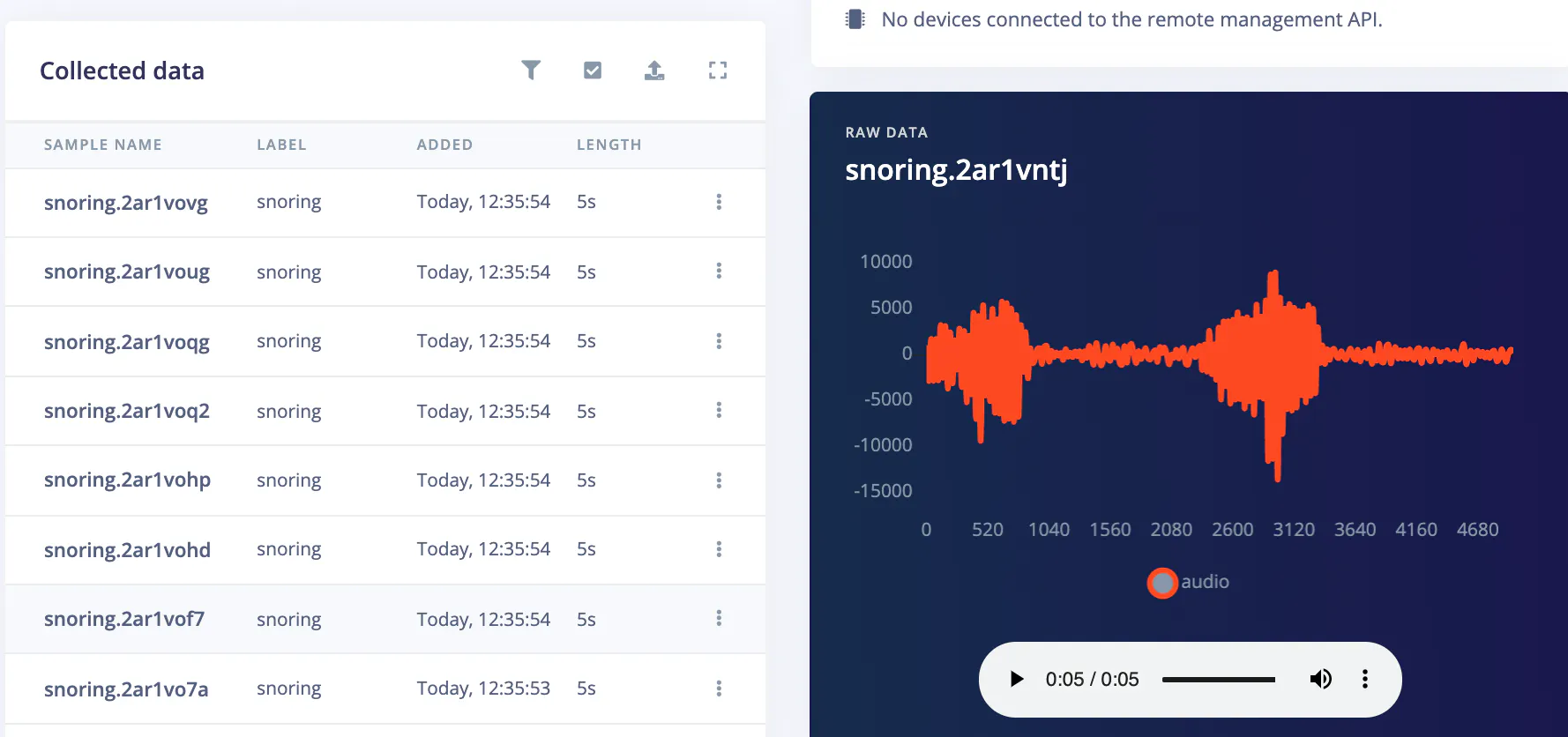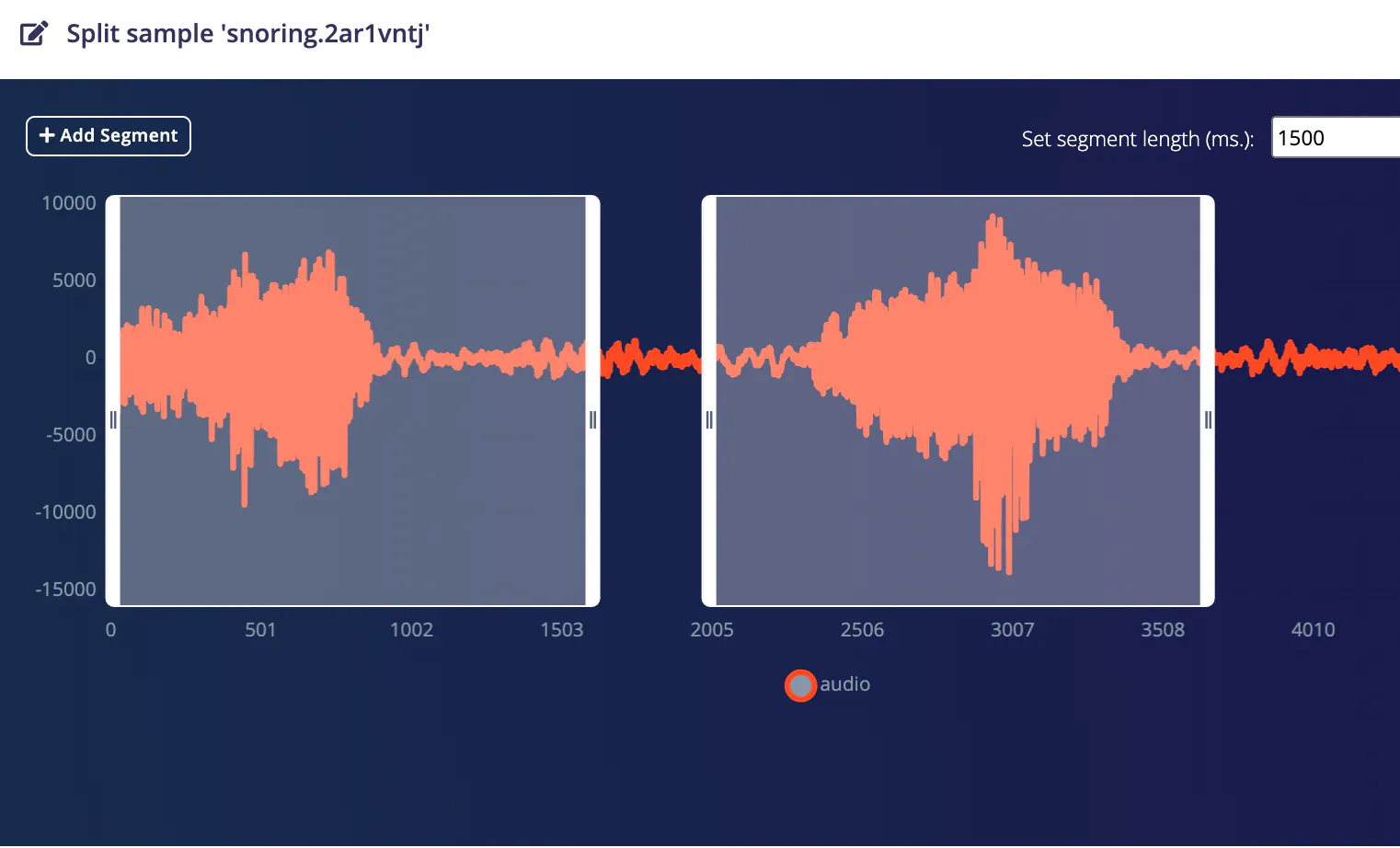import json import requests import logging import threading API_KEY = " Keys" projectId = "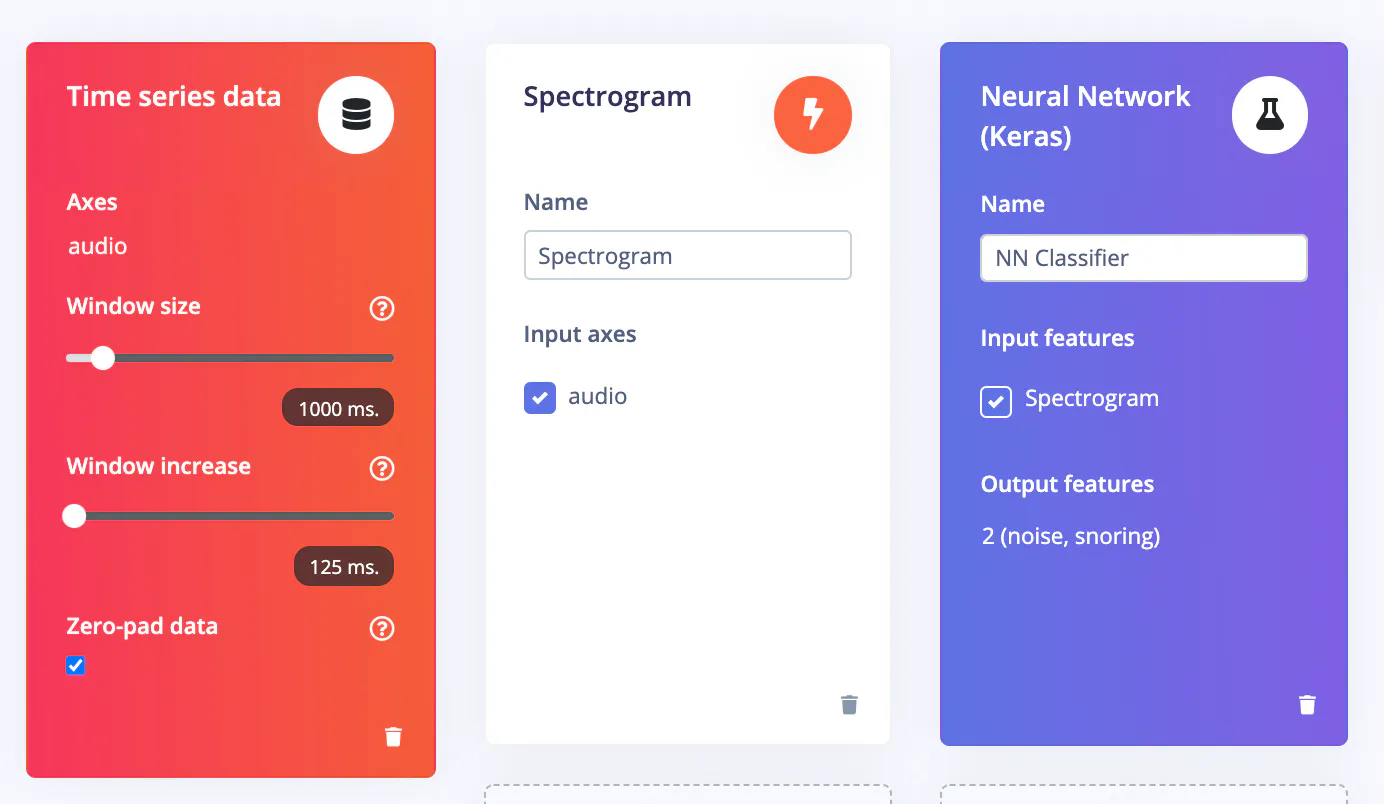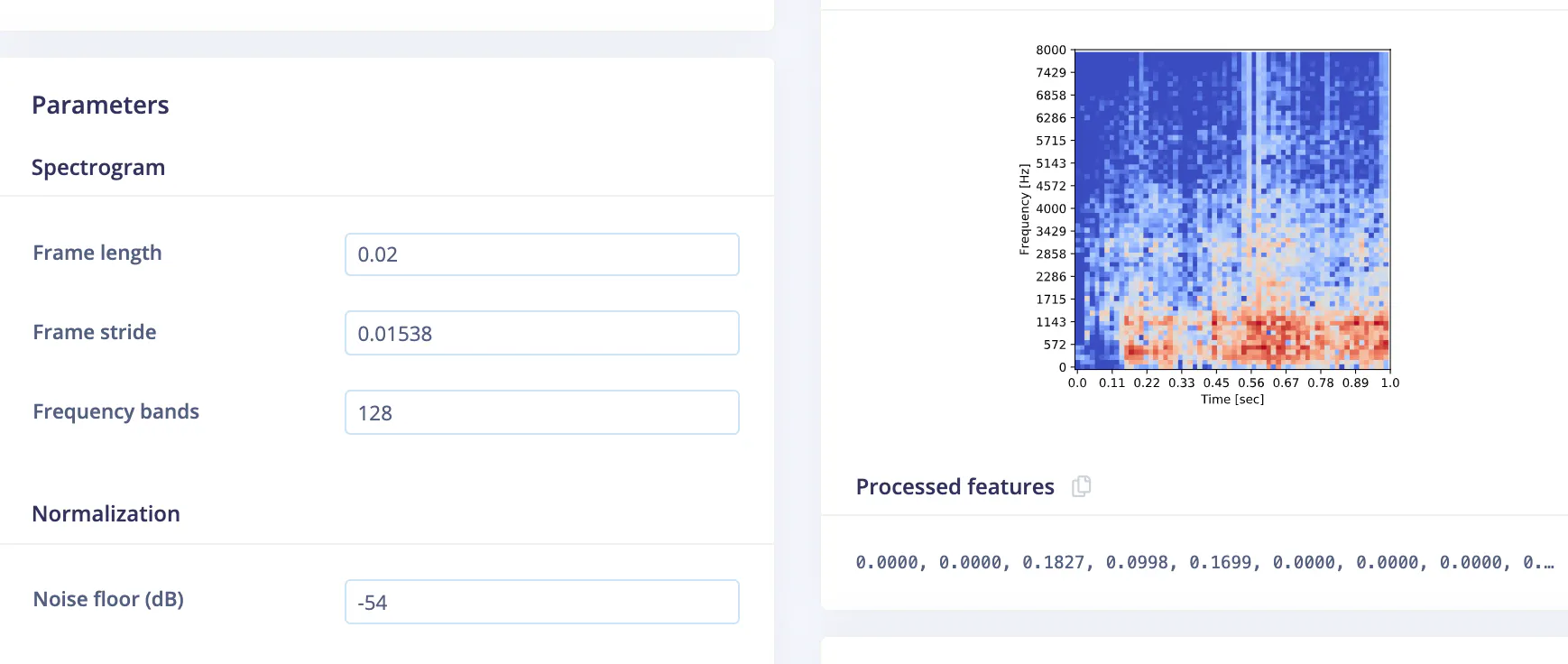```import tensorflow as tf from tensorflow.keras.models import Sequential from tensorflow.keras.layers import Reshape, Conv2D, Flatten, ReLU, Dropout, MaxPooling2D, Dense from tensorflow.keras.optimizers.schedules import InverseTimeDecay from tensorflow.keras.optimizers import Adam from tensorflow.keras.layers.experimental import preprocessing``` sys.path.append('./resources/libraries') import ei_tensorflow.training channels = 1 columns = 65 rows = int(input_length / (columns * channels)) norm_layer = preprocessing.Normalization() norm_layer.adapt(train_dataset.map(lambda x, _: x)) # model architecture model = Sequential() model.add(Reshape((rows, columns, channels), input_shape=(input_length, ))) model.add(preprocessing.Resizing(24, 24, interpolation='nearest')) model.add(norm_layer) model.add(Conv2D(16, kernel_size=3)) #model.add(BatchNormalization()) #model.add(Activation('relu')) model.add(ReLU(6.0)) model.add(Conv2D(32, kernel_size=3)) #model.add(BatchNormalization()) #model.add(Activation('relu')) model.add(ReLU(6.0)) model.add(MaxPooling2D(pool_size=2, strides=2, padding='same')) model.add(Dropout(0.7)) model.add(Flatten()) model.add(Dense(64)) #model.add(BatchNormalization()) #model.add(Activation('relu')) model.add(ReLU(6.0)) #model.add(Dropout(0.50)) model.add(Dense(32)) #model.add(BatchNormalization()) #model.add(Activation('relu')) model.add(ReLU(6.0)) #model.add(Dropout(0.50)) model.add(Dense(classes, activation='softmax', name='y_pred')) BATCH_SIZE = 64 lr_schedule = InverseTimeDecay(   0.0005,   decay_steps=train_sample_count//BATCH_SIZE*15,   decay_rate=1,   staircase=False) def get_optimizer():   return Adam(lr_schedule) train_dataset = train_dataset.batch(BATCH_SIZE, drop_remainder=False) validation_dataset = validation_dataset.batch(BATCH_SIZE, drop_remainder=False) callbacks.append(BatchLoggerCallback(BATCH_SIZE, train_sample_count)) # train the neural network model.compile(loss='categorical_crossentropy', optimizer=get_optimizer(), metrics=['accuracy']) print(model.summary()) model.fit(train_dataset, epochs=70, validation_data=validation_dataset, verbose=2, callbacks=callbacks)

 Model: "sequential" _________________________________________________________________  Layer (type)                 Output Shape              Param #     =================================================================  reshape (Reshape)            (None, 64, 65, 1)         0           _________________________________________________________________  resizing (Resizing)          (None, 24, 24, 1)         0           _________________________________________________________________  normalization (Normalization (None, 24, 24, 1)         3           _________________________________________________________________  conv2d (Conv2D)              (None, 22, 22, 16)        160         _________________________________________________________________  re_lu (ReLU)                 (None, 22, 22, 16)        0           _________________________________________________________________  conv2d_1 (Conv2D)            (None, 20, 20, 32)        4640        _________________________________________________________________  re_lu_1 (ReLU)               (None, 20, 20, 32)        0           _________________________________________________________________  max_pooling2d (MaxPooling2D) (None, 10, 10, 32)        0           _________________________________________________________________  dropout (Dropout)            (None, 10, 10, 32)        0           _________________________________________________________________  flatten (Flatten)            (None, 3200)              0           _________________________________________________________________  dense (Dense)                (None, 64)                204864      _________________________________________________________________  re_lu_2 (ReLU)               (None, 64)                0           _________________________________________________________________  dense_1 (Dense)              (None, 32)                2080        _________________________________________________________________  re_lu_3 (ReLU)               (None, 32)                0           _________________________________________________________________  y_pred (Dense)               (None, 2)                 66          =================================================================  Total params: 211,813  Trainable params: 211,810  Non-trainable params: 3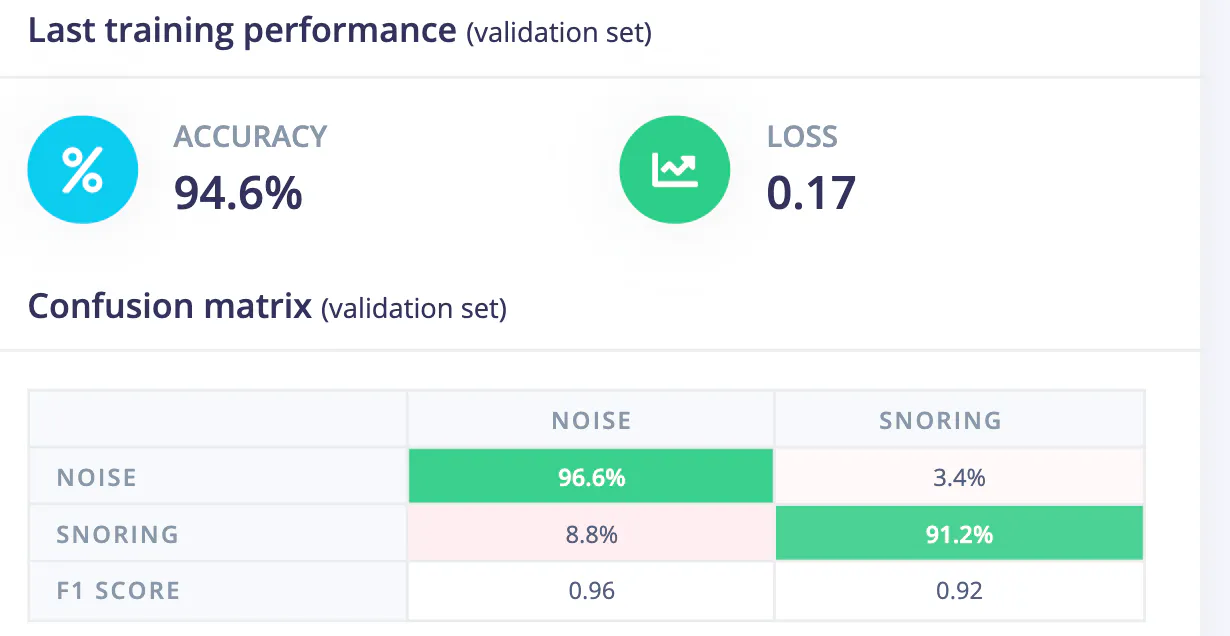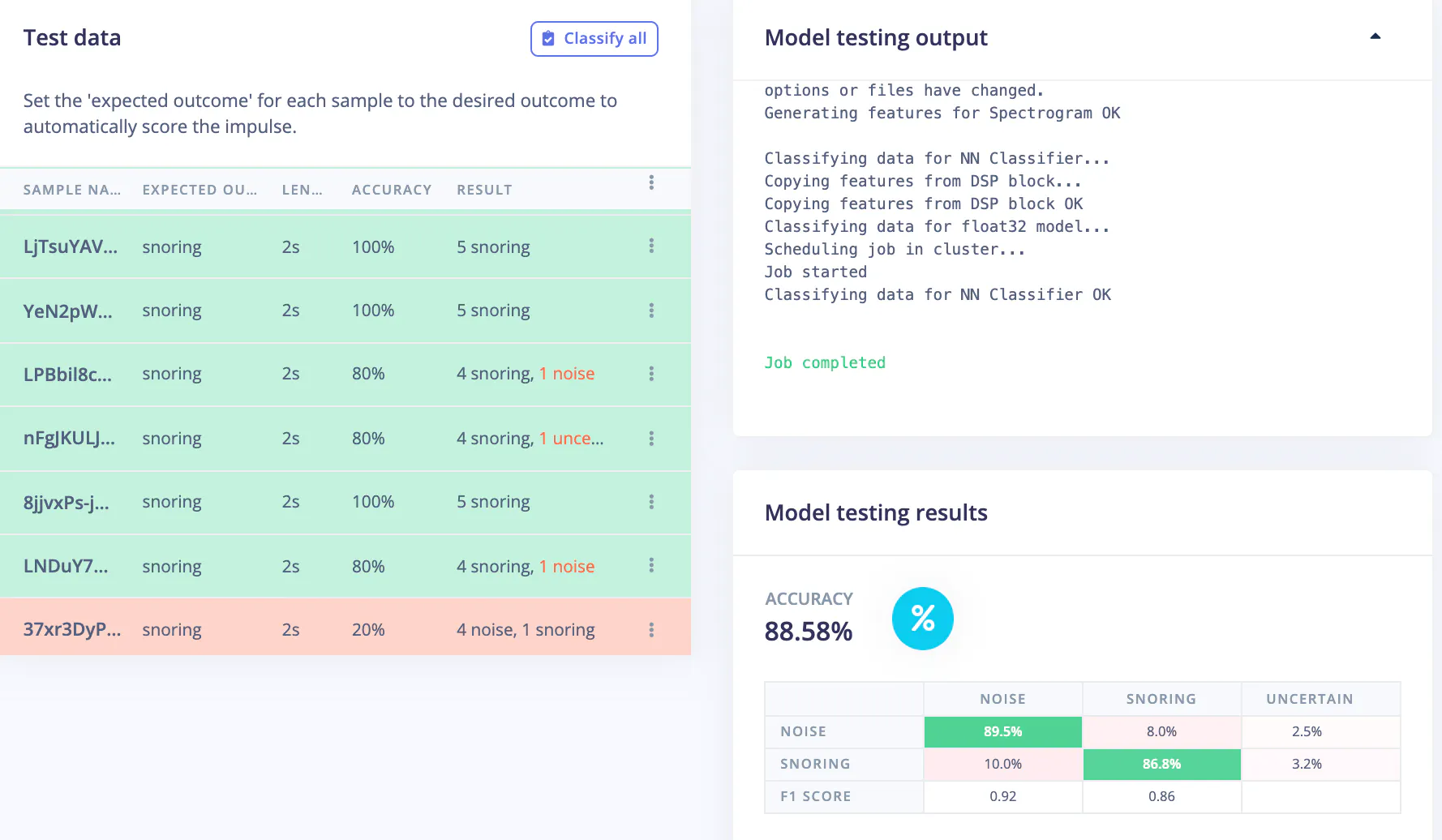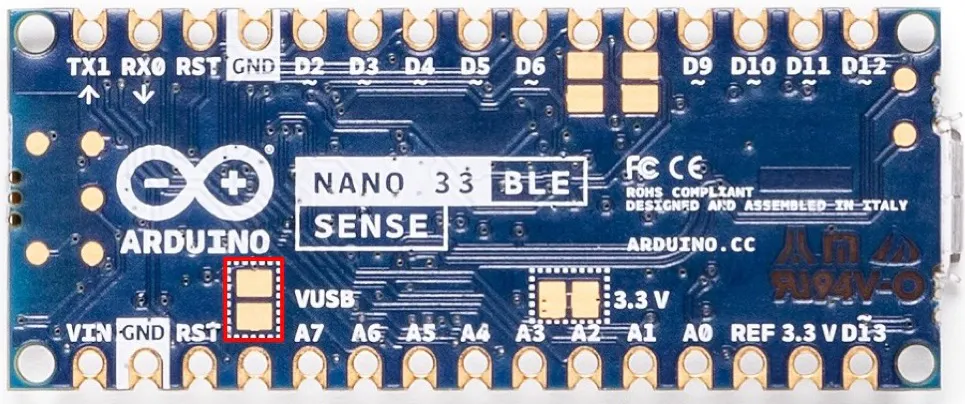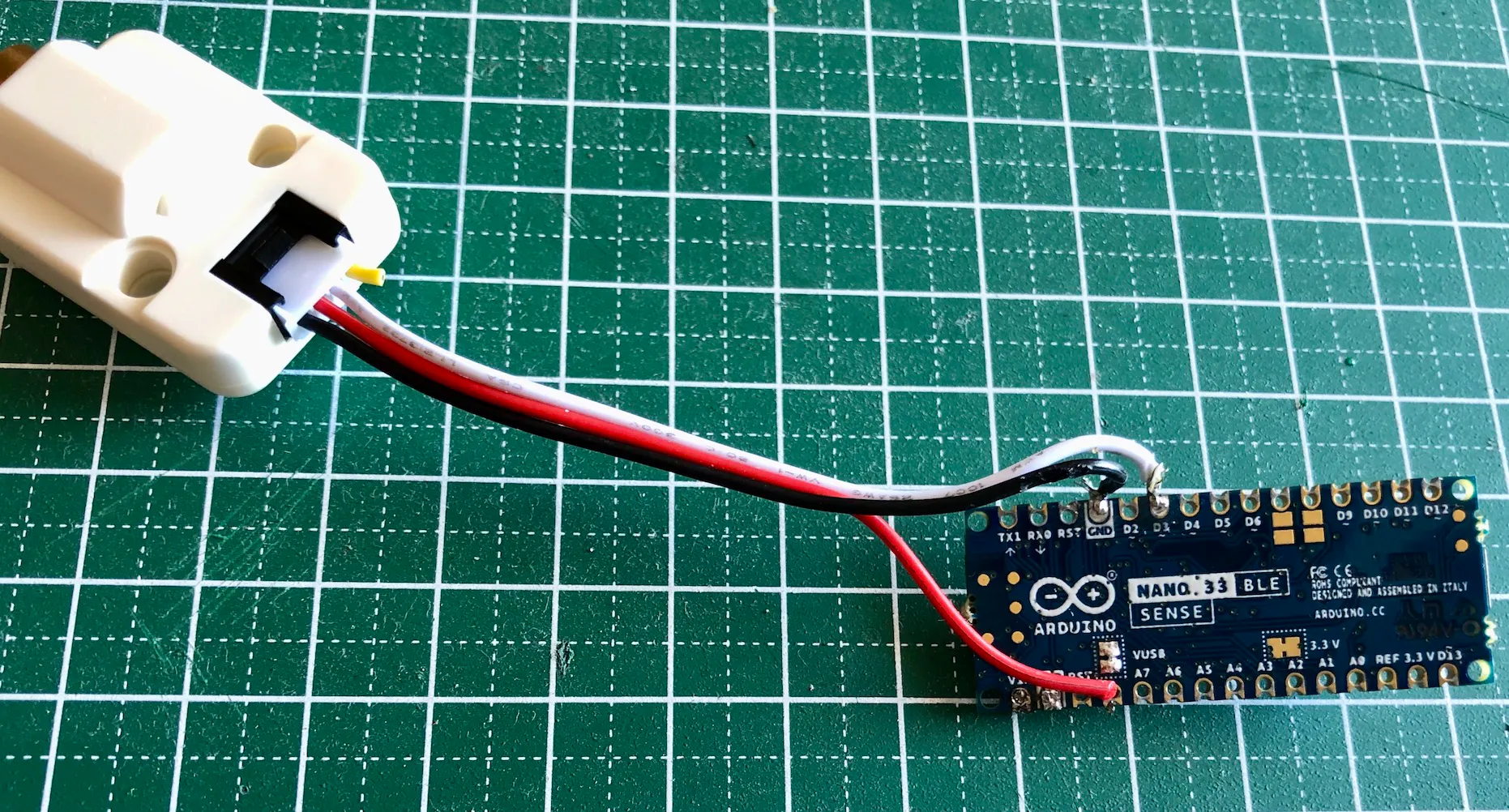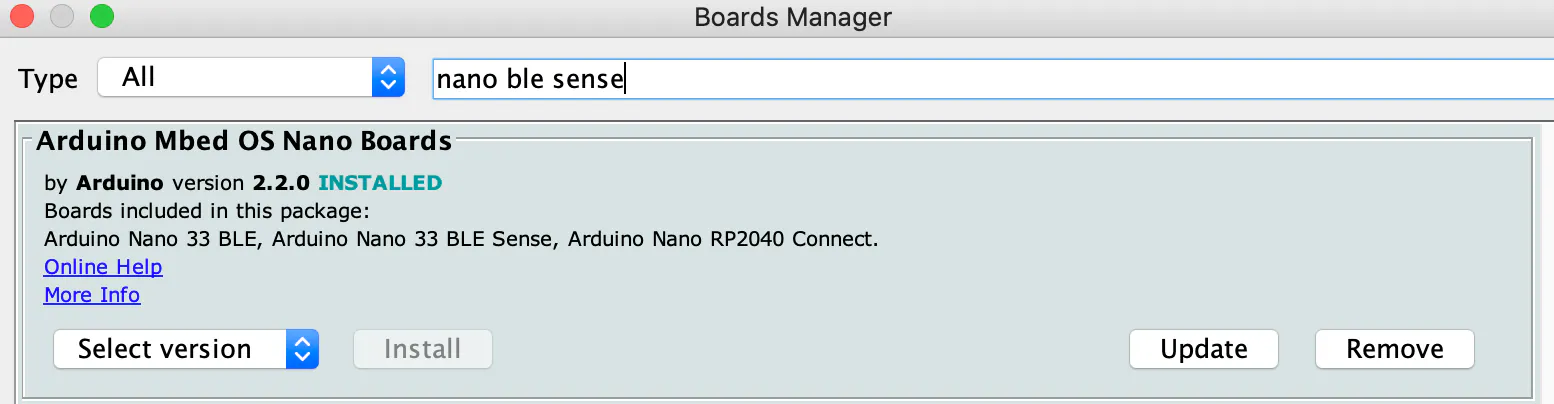\$ git clone https://github.com/metanav/Snoring_Guardian.git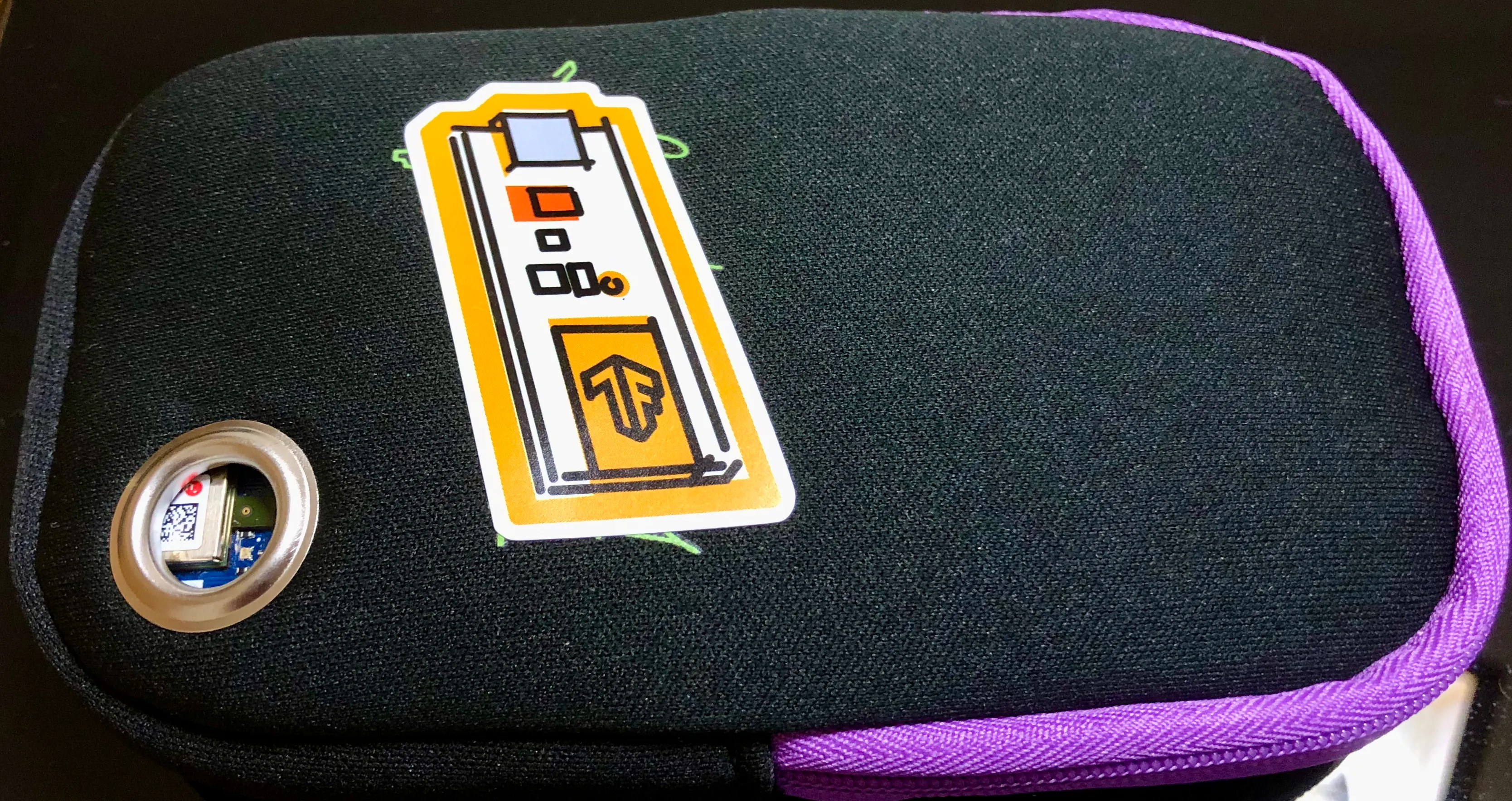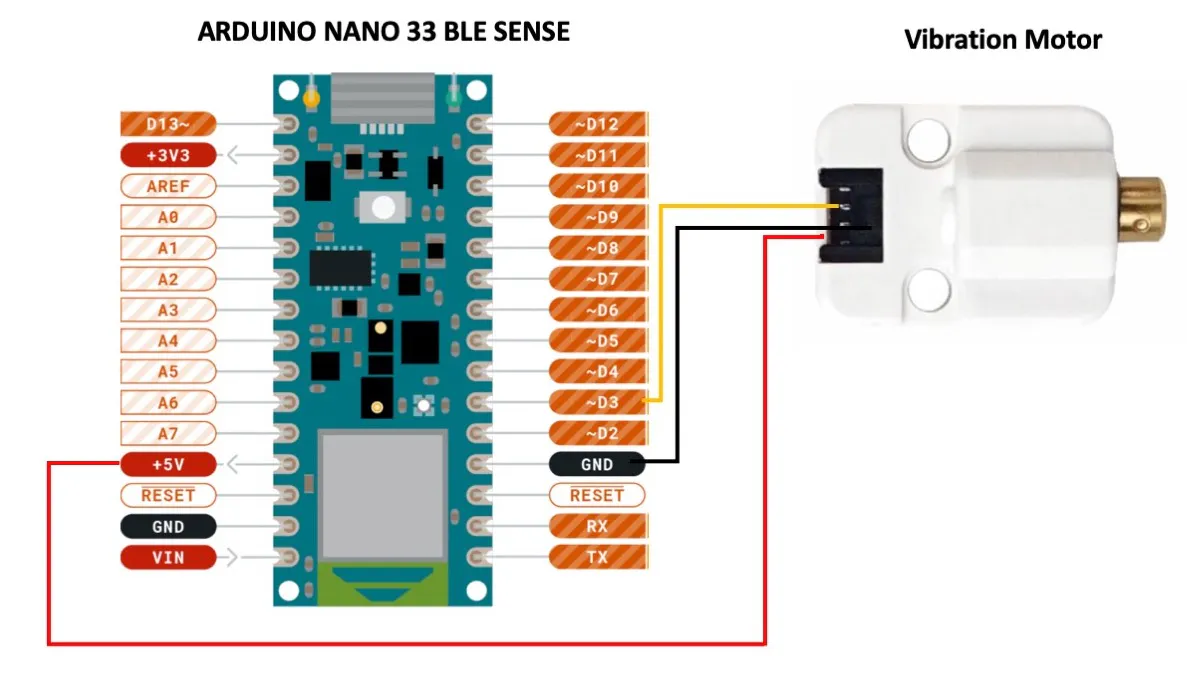加入微信技术交流群关注与非网服务号加入电路城 QQ 交流群